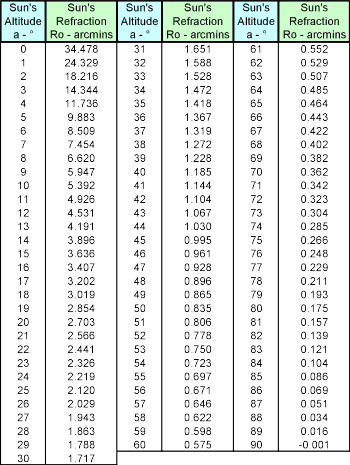The Sundial Primer created by Carl SabanskiThe Sundial Primer Index
 Refraction of Light Refraction: the "bending" of light at the interface of two materials of different refractive indices. It accounts for the focusing action of lenses. In dialling, use is made of refraction in dials which use a clear liquid in a solid cup to compress the hour lines, or which use a cylindrical lens to focus sunlight onto a curved dial plate. Atmospheric refraction (due to the curve of the Earth's surface and the variation of atmospheric density with height, in turn dependent on meteorological conditions) is the effect which makes the Sun (or other celestial body) look slightly higher in the sky than its true astronomical position. It is only significant when the Sun is within a few degrees of the horizon. At 0° altitude, the bending is equivalent to approximately 34 arc-minutes, so that it is possible to see the Sun when it has actually just sunk below the horizon. This effect is not known to be included in practical sundials.Refractive index (µ, MU): of a medium (or its index of refraction) is defined as the ratio of the speed of light in a vacuum to its speed in the medium. The Sun's refraction can be calculated using the following equation:Ro = 1 / tan [a + 7.31 / (a + 4.4)] where "a" is the Sun's altitude. Ro is the refraction in arc-minutes for a temperature of 10°C and an atmospheric pressure of 1010 millibars (mb).  For other conditions, a multiplying factor 0.28P/T is required, where P is the pressure in mb and T is the temperature in degrees kelvin (= temp in °C + 273). Table 1 shows how the Sun's refraction varies with its altitude.Table 1: Sun's RefractionThere are some sundials that are submerged in a liquid, water for example, to compress the hour lines. They are called refraction sundials. These sundials use the refractive property of the liquid to bend the rays of sunlight as they enter the liquid. To see this effect hold a straw vertically in a glass of water. As you move the straw away from the vertical position look at it from the top and also through the side of the glass.  Refraction is the bending of light that takes place at a boundary between two mediums having different indices of refraction. The boundary is where one medium meets another medium.  Refraction is due to a change in the speed of light as it passes from one medium to another.  Figure 1 illustrates this concept.Figure 1: Refraction of Light (CAD) The angles of incidence and refraction are measured relative to a line perpendicular to the boundary between the mediums called the normal. The mediums that the light passes from and to are transparent. The light will bend based on a relationship called Snell's Law.µi sin(Ai) = µr sin(Ar)µi is the refractive index of the medium that the light is leaving. Ai is the angle of incidence between the incident ray and the normal.. µr is the refractive index of the medium that the light is entering. Ar is the angle of refraction between the refracted ray and the normal.The incident and refracted rays are on opposite sides of the normal at the point of incidence. The incident ray, the normal, and the refracted ray are coplanar. As the light slows down it will bend toward the normal line and as it speeds up it will bend away.The absolute index of refraction for a given medium is defined as: µ = c/v where c is the speed of light in a vacuum and v is the speed of light in the medium. The refractive index of a vacuum is 1.Let us examine a refraction sundial where the sunlight passes from air into water. The refractive index of air is 1.0002926 and that of water (at 20°C) is 1.33335.  Figure 2 illustrates what happens as light passes into water with a change in the angle of incidence. At the critical angle, in this case 48.609°, the angle of refraction becomes ±90°. Light is no longer transmitted into the water but is totally reflected.Figure 2: Refraction of Light - Air to Water Figure 3 illustrates a horizontal sundial that is submerged in water and the effects of refraction on the hour lines.Figure 3: Underwater Horizontal Sundial (ZW2000/CAD) There are other sundials, such as the window, reflection (ceiling) and stained glass, that can be affected by the refraction of glass. The stained glass sundial is only affected if it is placed behind a clear glass window, which is usually done to protect it. The window and reflection dials require that the sun's rays pass through the window. The "Window Sundial" page discusses the affect on sunlight as it passes through glass.